## Completion Of Incomplete Pattern

#### Completion Of Incomplete Pattern

1. Which answer figure completes the form in question figure?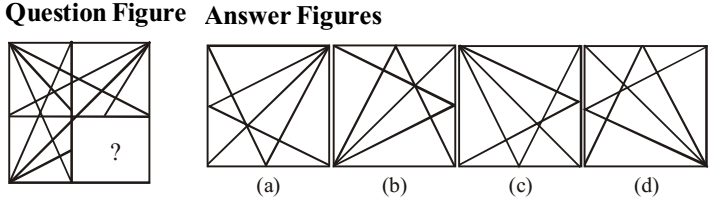1. As we can see that complete figure form will be :-
Option (d) will complete the question figure.

##### Correct Option: D

As we can see that complete figure form will be :-
Option (d) will complete the question figure.So , required answer is figure ( 4 ) .

1. Which answer figure will complete the pattern in the question figure?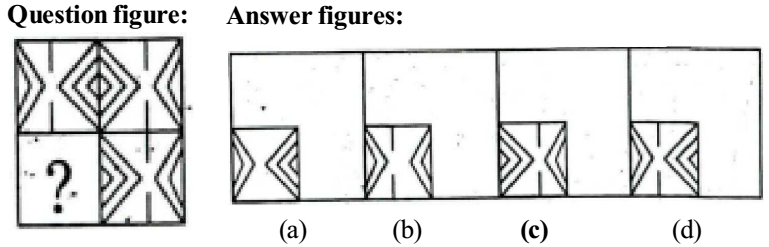1. We follow the given pattern and complete form will be :-
Figure ( d ) will be complete form of given figure .

##### Correct Option: D

We follow the given pattern and complete form will be :-
Figure ( d ) will be complete form of given figure .

1. Which answer figure will complete the pattern in the question figure?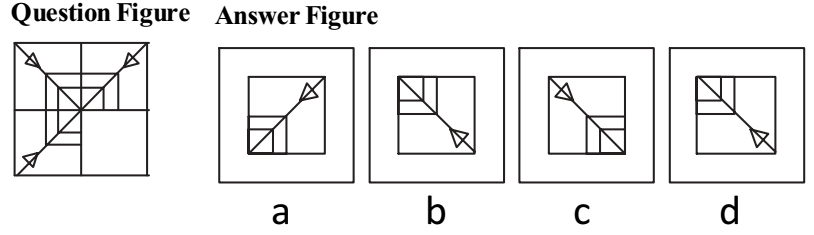1. NA

##### Correct Option: B

NA

1. Which answer figure will complete the pattern in the question figure?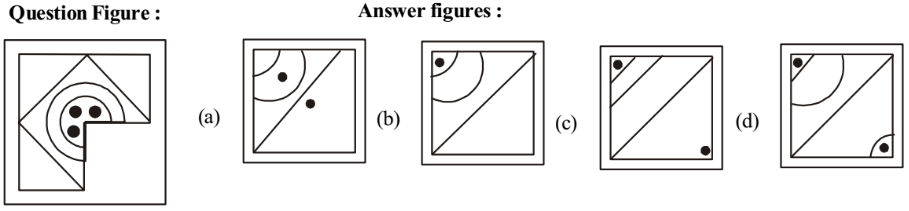1. Dot is only in first circle so figure (b) will complete the picture in the question figure.

##### Correct Option: B

Dot is only in first circle so figure (b) will complete the picture in the question figure.

1. Which answer figure will complete the pattern in the question figure?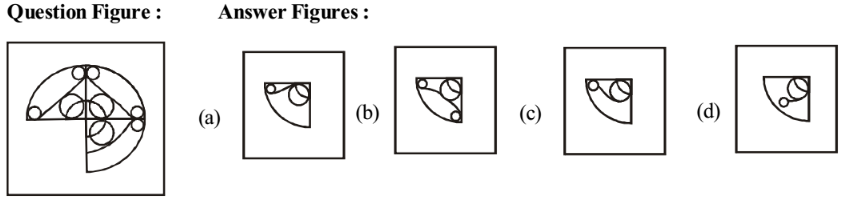1. NA

NA